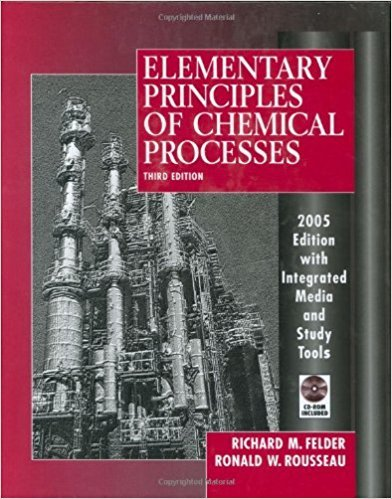×
Get Full Access to Elementary Principles Of Chemical Processes - 3 Edition - Chapter 6 - Problem 6.68
Get Full Access to Elementary Principles Of Chemical Processes - 3 Edition - Chapter 6 - Problem 6.68

×

# Vapor-liquid equilibrium data for mixtures of acetone (A)ISBN: 9780471687573 143

## Solution for problem 6.68 Chapter 6

Elementary Principles of Chemical Processes | 3rd Edition

• Textbook Solutions
• 2901 Step-by-step solutions solved by professors and subject experts
• Get 24/7 help from StudySoup virtual teaching assistantsElementary Principles of Chemical Processes | 3rd Edition

4 5 1 356 Reviews
31
5
Problem 6.68

Vapor-liquid equilibrium data for mixtures of acetone (A) and ethanol at 1 atm are given in the following table: Encyclopedia Equipment evaporator 299 T(C) 78.3 67.3 65.9 63.6 61.8 60.4 59.1 58.0 I : 57.0 56.1 XA 0.000 0.250 0.300 0.400 0.500 0.600 0.700 0.800 I 0.900 1.000 YA 0.000 0.478 0.524 0.605 0.674 0.739 0.802 0.865 I 0.929 1.000 (a) Use the given data to construct a Txy diagram for this system. (b) A thermocouple inserted into a two-phase mixture of acetone and ethanol at equilibrium reads 62.1C. The system pressure is 1 atm. Use the Txy diagram to estimate the mole fractions of acetone in the liquid and vapor phases. (c) An equimolar mixture of acetone and ethanol is fed to an evacuated vessel and allowed to come to equilibrium at 65C and 1.00 atm absolute. Estimate (i) the molar compositions of each phase, (ii) the percentage of the total moles in the vessel that are in the vapor phase. and (iii) the percentage of the vessel volume occupied by the vapor phase. (d) A liquid mixture containing 40.0 mole% acetone and 60.0 mole% ethanol is fed to a continuous flash evaporator. Vapor and product streams leave the unit in equilibrium at 1.00 atm. The molar flow rate of the vapor product stream is 20% of the molar flow rate of the feed stream. Estimate the operating temperature of the evaporator and the compositions of the liquid and vapor product streams. (e) Use Raoult's law to estimate the bubble-point temperature and vapor composition in equilibrium with an equimolar liquid mixture of acetone and ethanol. Calculate the percentage errors in the estimated values of h p and y. Propose a reason why Raoult's law produces poor estimates for this system. (Suggestion: Consider the molecular structure of the two components.)

Step-by-Step Solution:
Step 1 of 3

CHEM 142 – Craig (10/2/17) LECTURE 1.2: Atomic Emission Spectra and Bohr’s Model Reading Blackbody Radiation  heated object will emit energy as EM radiation  Max Planck: experiments on light emitted from solid heated to incandescence  as temperature increases, intensity increases, peak λ shifts to shorter wavelengths  Planck also worked on mathematical model of characteristic BB radiation curve Energy Quantization  energy of particular frequency is lost in integer values nh ­34  ∆E = ν (∆E = energy change, n = integer, ν = frequency, h = 6.626 × 10 J∙s  blackbody system can absorb/emit energy of given frequency only in discrete amounts h

Step 2 of 3

Step 3 of 3

##### ISBN: 9780471687573

Elementary Principles of Chemical Processes was written by and is associated to the ISBN: 9780471687573. The answer to “Vapor-liquid equilibrium data for mixtures of acetone (A) and ethanol at 1 atm are given in the following table: Encyclopedia Equipment evaporator 299 T(C) 78.3 67.3 65.9 63.6 61.8 60.4 59.1 58.0 I : 57.0 56.1 XA 0.000 0.250 0.300 0.400 0.500 0.600 0.700 0.800 I 0.900 1.000 YA 0.000 0.478 0.524 0.605 0.674 0.739 0.802 0.865 I 0.929 1.000 (a) Use the given data to construct a Txy diagram for this system. (b) A thermocouple inserted into a two-phase mixture of acetone and ethanol at equilibrium reads 62.1C. The system pressure is 1 atm. Use the Txy diagram to estimate the mole fractions of acetone in the liquid and vapor phases. (c) An equimolar mixture of acetone and ethanol is fed to an evacuated vessel and allowed to come to equilibrium at 65C and 1.00 atm absolute. Estimate (i) the molar compositions of each phase, (ii) the percentage of the total moles in the vessel that are in the vapor phase. and (iii) the percentage of the vessel volume occupied by the vapor phase. (d) A liquid mixture containing 40.0 mole% acetone and 60.0 mole% ethanol is fed to a continuous flash evaporator. Vapor and product streams leave the unit in equilibrium at 1.00 atm. The molar flow rate of the vapor product stream is 20% of the molar flow rate of the feed stream. Estimate the operating temperature of the evaporator and the compositions of the liquid and vapor product streams. (e) Use Raoult's law to estimate the bubble-point temperature and vapor composition in equilibrium with an equimolar liquid mixture of acetone and ethanol. Calculate the percentage errors in the estimated values of h p and y. Propose a reason why Raoult's law produces poor estimates for this system. (Suggestion: Consider the molecular structure of the two components.)” is broken down into a number of easy to follow steps, and 300 words. This textbook survival guide was created for the textbook: Elementary Principles of Chemical Processes, edition: 3. The full step-by-step solution to problem: 6.68 from chapter: 6 was answered by , our top Chemistry solution expert on 11/15/17, 02:42PM. This full solution covers the following key subjects: vapor, acetone, ethanol, liquid, Equilibrium. This expansive textbook survival guide covers 13 chapters, and 710 solutions. Since the solution to 6.68 from 6 chapter was answered, more than 251 students have viewed the full step-by-step answer.

Unlock Textbook Solution